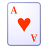# QlikView App Dev

Discussion Board for collaboration related to QlikView App Development.

Announcements
Action-Packed Learning Awaits! QlikWorld 2023. April 17 - 20 in Las Vegas: REGISTER NOW
cancel
Showing results for
Did you mean:Creator

## Sum AGGR divided by

Hi experts,

on a dynamic straight table, in the column "Dim3 Value" I obtain the following result with "sum(aggr(Value,Dim1,Dim3))"

Dim 1Dim 2Dim 3Dim3 Value
TOTAL: 140
ABE0
ABF0
ABG0
ACE0
ACF0
ACG0

But I would like to obtain this:

Dim 1Dim 2Dim 3Dim3 Value
Total: 140
ABE84
ABF15
ABG41
ACE84
ACF15
ACG41

I tried to do this: "sum(aggr(Value,Dim1,Dim3,Dim2))/count (distinct Dim2)", it work only if the DIM1 is only one selected value (in my example the selected value is "A") but if i select more than one DIM1 my qlikview desktop freezes my laptop.

1 Solution

Accepted SolutionsAnonymous
Not applicable

perhaps:

sum(aggr(nodistinct Value,Dim1,Dim3))

or

sum(total <Dim1,Dim3> value)

6 RepliesSpecialist

Can you provide sample data?? or a sample qvw??Champion III

Try this? Else can you share a sample ?

= sum(aggr(Sum(Value), Dim1, Dim2,Dim3)) / count (distinct Dim2)Anonymous
Not applicable

perhaps:

sum(aggr(nodistinct Value,Dim1,Dim3))

or

sum(total <Dim1,Dim3> value)MVP

May be try this:

Sum(TOTAL <Dim1, Dim3> Value)

or

Sum(TOTAL <Dim1, Dim3> Aggr(Value, Dim1, Dim2, Dim3))Specialist